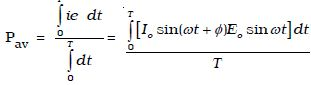# Explain with Equation: Power in an AC Circuit

In an a.c circuit the current and emf vary continuously with time. Therefore power at a given instant of time is calculated and then its mean is taken over a complete cycle. Thus, we define instantaneous power of an a.c. circuit as the product of the instantaneous emf and the instantaneous current flowing through it.

The instantaneous value of emf and current is given by

e = E0 sin ωt

i = I0 sin (ωt + φ)

where φ is the phase difference between the emf and current in an a.c circuit

The average power consumed over one complete cycle isOn simplification, we obtain

Pav = (E0 I0) /2 cos φ

Pav = E0/√2 . I0√2 cos φ= Erms . Irms φ

Pav = apparent power × power factor

where Apparent power = Erms Irms and power factor = cos φ

The average power of an ac circuit is also called the true power of the circuit.